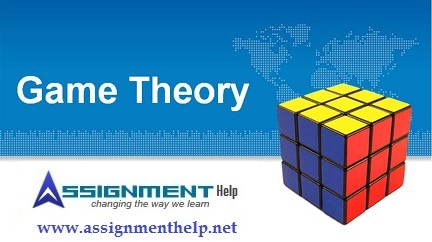Game theory is a well-known as mathematical method for analyzing calculated circumstances, such as in games, where a person’s success based upon the choices of others. In other words, it is “the study of mathematical models of conflict and cooperation between intelligent rational decision-makers. The subject first addressed zero-sum games, such that one person’s gains exactly equal net losses of the other participants.Game theory has found its applications in numerous fields such as Economics, Social Science, Political Science, and Evolutionary Biology. Game theory is now finding its applications in computer science. The nature of computing is changing because of success of internet and the revolution in information technology.

What is game theory?
Game theory is the formal study of conflict and cooperation. Game theoretic concepts apply whenever the actions of several agents are interdependent. These agents may be individuals, groups, firms, or any combination of these.

Game theory can be regarded as a multi-agent decision problem. Which means there are many people contending for limited rewards/payoffs. They have to make certain moves on which their payoff depends. These people have to follow certain rules while making these moves. Each player is supposed to behave rationally.

Nash equilibrium: A Nash equilibrium, also called strategic equilibrium, is a list of strategies, one for each player, which has the property that no player can unilaterally change his strategy and get a better payoff.

Payoff: A payoff is a number, also called utility, that reflects the desirability of an outcome to a player, for whatever reason. When the outcome is random, payoffs are usually weighted with their probabilities. The expected payoff incorporates the player’s attitude towards risk.

Rationality: In the language of game theory rationality implies that each player tries to maximize his/her payoff irrespective to what other players are doing. In essence each player has to decide a set of moves which are in accordance with the rules of the game and which maximize his/her rewards.

Zero-sum game: A game is said to be zero-sum if for any outcome, the sum of the payoffs to all players is zero. In a two-player zero-sum game, one player’s gain is the other player’s loss, so their
interests are diametrically opposed.

Game Theory can be classified in two branches as follows: —

1. Non co-operative game theory: In this case the players work independently without assuming anything about what other players are doing.
2. Co-operative game theory: Here players may co-operate with one another.

What is a game?

A game has the following attributes: —

1. Set of players              D = { Pi | 1 < = i <= n }
2. Set of rules                 R
3. Set of Strategies          Si for each player Pi
4. Set of Outcomes          O
5. Payoff                        ui (o) for each player i and for each outcome                                     o e O

We cover all major topics like algebra, calculus, geometry, mathematical logic, differential equations, number theory, probability and statistics, computing, economics, game theory and operations research where you can take guidance/help from our expert tutors. You can understand this game theory by following e.g. Prisoner’s dilemma (a classic example of game theory), Dollar auction, Assurance game, Signaling game, War of attrition, and Coordination game.

In game theory, nothing is fixed!!

If you have any questions about our assignment help services – don’t hesitate to Contact Us. Please feel free to call us for assistance at any time (24*7).

Suggested Books:

Binmore, Ken (1991), Fun and Games: A Text on Game Theory. D. C. Heath, Lexington, MA.

Gibbons, Robert (1992), Game Theory for Applied Economists. Princeton University Press, Princeton, NJ.